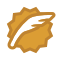# Box of Bricks

Time Limit: 2000/1000 MS (Java/Others)    Memory Limit: 65536/32768 K (Java/Others)
Total Submission(s): 3669    Accepted Submission(s): 1664

Problem Description
Little Bob likes playing with his box of bricks. He puts the bricks one upon another and builds stacks of different height. Look, I've built a wall!'', he tells his older sister Alice. Nah, you should make all stacks the same height. Then you would have a real wall.'', she retorts. After a little con- sideration, Bob sees that she is right. So he sets out to rearrange the bricks, one by one, such that all stacks are the same height afterwards. But since Bob is lazy he wants to do this with the minimum number of bricks moved. Can you help?Input
The input consists of several data sets. Each set begins with a line containing the number n of stacks Bob has built. The next line contains n numbers, the heights hi of the n stacks. You may assume 1 <= n <= 50 and 1 <= hi <= 100.

The total number of bricks will be divisible by the number of stacks. Thus, it is always possible to rearrange the bricks such that all stacks have the same height.

The input is terminated by a set starting with n = 0. This set should not be processed.

Output
For each set, first print the number of the set, as shown in the sample output. Then print the line The minimum number of moves is k.'', where k is the minimum number of bricks that have to be moved in order to make all the stacks the same height.

Output a blank line after each set.

Sample Input
6 5 2 4 1 7 5 0

Sample Output
Set #1 The minimum number of moves is 5.

具体为什么这样做 ，还有待思考

#include<iostream>
#include<cmath>
using namespace std;

int main()
{
int a;
int i, n;
int m = 1;
while(cin>>n && n)
{
int sum = 0;
for(i = 0; i < n; i++)
{
cin>>a[i];
sum += a[i];
}
sum /= n;
int ans = 0;
for(i = 0; i < n; i++)
{
ans += abs(sum - a[i]);
}
ans /= 2;
cout<<"Set"<<" "<<"#"<<m<<endl;
cout<<"The minimum number of moves is"<<" "<<ans<<"."<<endl;
cout<<endl;
m++;
}

return 0;
}


wangxiaomingCSDN认证博客专家 架构 Spring Boot Redis

09-116546

#### 无法连接到sql数据库,在与sql server建立连接时出现与网络,Microsoft SQL Server，错误: 132611-09278

#### hdu1326-简单数学题

05-1125

#### zoj 2151 所有小于平均数与平均数的差的和

12-05606

#### hdu 1326

08-10152

#### HDU1326:Box of Bricks

04-14135

#### 杭电1326

09-241695

#### Microsoft SQL Server，错误: 1326

01-01525

#### SQL Server连接错误1326©️2020 CSDN 皮肤主题: Age of Ai 设计师: meimeiellie点击重新获取扫码支付1.余额是钱包充值的虚拟货币，按照1:1的比例进行支付金额的抵扣。
2.余额无法直接购买下载，可以购买VIP、C币套餐、付费专栏及课程。余额充值